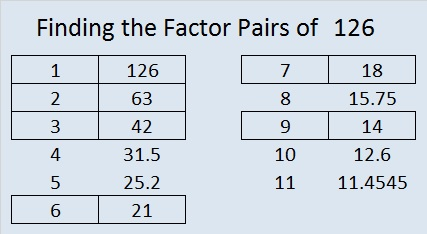# 126 and Level 5

126 is a composite number. 126 = 1 x 126, 2 x 63, 3 x 42, 6 x 21, 7 x 18, or 9 x 14. Factors of 126: 1, 2, 3, 6, 7, 9, 14, 18, 21, 42, 63, 126. Prime factorization: 126 = 2 x 3 x 3 x 7, which can also be written 2 x 3² x 7.Thinking process using divisibility tricks to find the factor pairs of 126 quickly:

√126 is irrational and approximately equal to 11.22. Every factor pair of 126 will have one factor less than 11.22 and one factor greater than 11.22, and we will find both factors in each pair at the same time. The following integers are less than 11.22. Are they factors of 126?

1. Yes, all whole numbers are divisible by 1, so 1 x 126 = 126.
2. Yes, 126 is an even number. 126 ÷ 2 = 63, so 2 x 63 = 126. (Since 63 is odd, 4 will not be a factor of 126.)
3. Yes, 1 + 2 + 6 = 9 which is divisible by both 3 and 9, so 126 is divisible by both 3 and 9, and 3 x 42 = 126.
4. No, the number formed from its last two digits, 26, isn’t divisible by 4, so 126 is not divisible by 4.
5. No, the last digit is not 0 or 5, so 126 is not divisible by 5.
6. Yes, 126 is divisible by both 2 and 3, so it is divisible by 6, and 6 x 21 = 126.
7. Yes, 63, 42, and 21 found above give this fact away. But also try the divisibility trick for 7 which requires us to split 126 into 12 and 6. We double 6 and subtract the double from 12. We get 12 – (2 x 6) = 12 – 12 = 0. Since 0 is divisible by 7, 126 is also divisible by 7, and 7 x 18 = 126.
8. No, since 126 isn’t divisible by 4, it is not divisible by any multiple of 4.
9. Yes, see 3 above. 126 is divisible by 9, and 9 x 14 = 126.
10. No, 126 doesn’t ends with a zero, so 10 is not a factor of 126.
11. No. I’ve typed the digits that are in an odd position in regular type and the digit in an even position in bold type: 126. To perform the divisibility trick for 11, we find the sum of the numbers typed in regular type (1 + 6  = 7) and the sum of the numbers typed in bold type (2) and subtract the smaller sum from the larger sum (7 – 2 = 5). If the difference were a multiple of 11, than the original number would also be a  multiple of 11 (and divisible by 11). Since 5 is not a multiple of 11, we know 126 is not divisible by 11.

From this thinking process we conclude that the factor pairs of 126 are 1 x 126, 2 x 63, 3 x 42, 6 x 21, 7 x 18, and 9 x 14.

126 is never a clue in the FIND THE FACTORS puzzles.

Today’s puzzle might be the most difficult level 5 puzzle I have ever published, so if you have never solved a level 5 or level 6 puzzle before, you probably don’t want to start with this one.Excel file of this week’s puzzles and last week’s factors: 12 Factors 2014-05-19## 2 thoughts on “126 and Level 5”

1.Steve Morris

I have learned a lot of useful factorization tricks here. Thank you! And I have worked out the solution, yay!

•ivasallay

I’m glad that I have been able to teach you a trick or two especially since I’ve learned so much reading your blog. Good for you working out the solution, too!

This site uses Akismet to reduce spam. Learn how your comment data is processed.